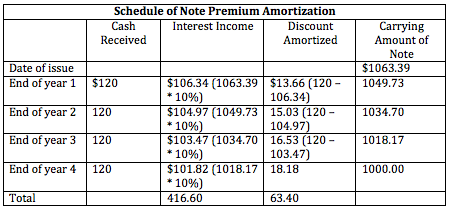Explore BrainMass

# Long-Term Notes Receivable

What changes as we move from short-term notes receivable to long-term notes receivable is the length of time to maturity and the importance of interest in measuring and accounting for the financial instrument.

The value of the instrument is measured and recognized on the balance sheet as the present value of the cash amounts that are expected to be collected from the notes receivable in the future, with the amounts discounted at the market rate of interest for loans with similar risk and other similar characteristics.

That being said, when the market interest rate is equal to the stated interest rate of the note receivable, its present value is simply equal to the face value of the note receivable. When the stated rate on the note differs from the market rate, the note is considered to be at either a discount or a premium, and this discount or premium must be amortized over the life of the note so that the interest recognized in each period is similar to the market rate of interest.

NOTES ISSUED AT FACE VALUE

Consider a \$1000 four-year note receivable with an interest payable of 10% annually. The market rate of interest for a similar note is 10% as well. Let's look at the timing and the amounts of the cash flows.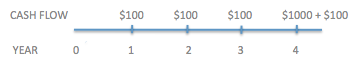We show below that the face value of \$100 is equal to the present value of the principal payment of the note as well as the present value of the interest annuity payments. To simplify things, we use the Present Value Factor for an Ordinary Annuity to calculate the present value of the annuity.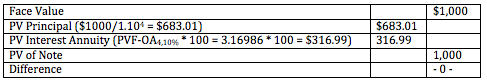In this case, the note would be recognized on the balance sheet at \$1000, and each year the interest payment of \$100 would be debited to cash and credited as interest income.

NOTES NOT ISSUED AT FACE VALUE

Zero-Interest-Bearing Notes Receivable

Consider the same note receivable for \$1,000 above with a 10% interest rate, but assume that not interest payments are payable during the life of the note.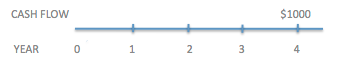We would discount the note receivable by the market interest rate to determine the present value of the note to be recorded on the books today. The difference between the face value of the note and the note receivable is called the discount and must be amortized over the life of the note receivable.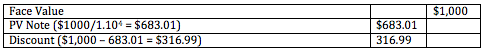At the end of each year, we would calculate the interest revenue earned from the note (even if no interest is paid), credit the amount of interest income earned, and debit the note receivable (or debit Note Discount, the contra note receivable account) to increase the carrying amount of the note. To illustrate these entries, we can make the following schedule for amortizing the note discount.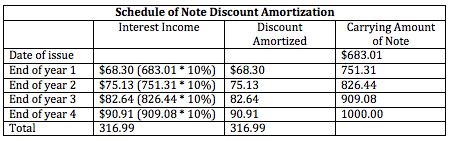Interest-Bearing Notes Receivable Issued at a Discount

Consider the same note we've been looking at before. This time though, while the market rate of interest is 10%, the interest payable on the note receivable is 8%.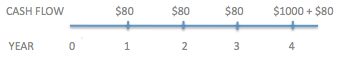Because the market rate of interest is more than the interest rate of the note, we say that the note is issued at a discount. The difference between the face value of the note and the present value of the cash flows from the note is called the dicsount, and it must be amortized over the life of the note.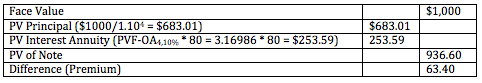We would record a carrying amount of the note today equal to its present value of \$936.60, and would amortize the discount over the life of the note. At the end of each year we would credit interest income with the amount of interest earned on the note, debit our bank account with the cash received as interest (\$80), and debit the difference to the discount on the note receivable to increase the carrying amount of the note. We can illustrate this in the following schedule.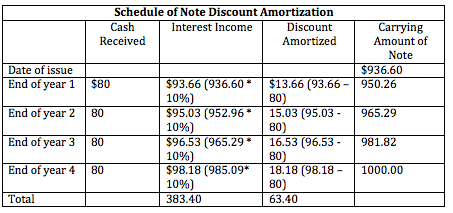Interest-Bearing Notes Receivable Issued at a Premium

Consider the same note we've been looking at before. This time though, while the market rate of interest is 10%, the interest payable on the note every year is equal to 12%.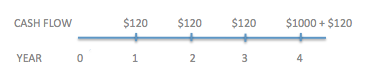Because the market rate of interest is less than the interest rate on the note, we say that the note is at a premium. The difference between the face value of the note and the present value of cash flows from the note is called the premium, and it must be amortized over the life of the note.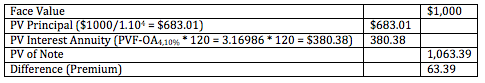We would record a carrying amount of the note today equal to its present value of \$1063.39, and would amortize this premium over the life of the note. At the end of each year we would debit our bank account with the cash received as interest (\$120), a portion of that equal to the interest payable equal to the market rate of interest would be credited as interest income, and the remainder would be credited to the carrying value of the note, or the premium. We can illustrate this in the following schedule.